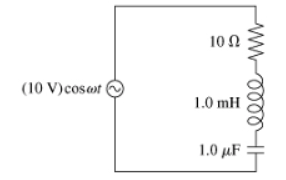# Problem: Part A. Find the Vr at resonance.Part B. Find the Vc at resonance,

###### FREE Expert Solution

Vr at resonance:

$\overline{){{\mathbf{V}}}_{{\mathbf{r}}}{\mathbf{=}}{\mathbf{i}}{\mathbf{R}}}$

Current:

$\overline{){\mathbf{i}}{\mathbf{=}}\frac{{\mathbf{V}}_{\mathbf{m}}}{\mathbf{Z}}}$

VC at resonance:

$\overline{){{\mathbf{V}}}_{{\mathbf{C}}}{\mathbf{=}}{{\mathbf{iX}}}_{{\mathbf{C}}}}$

XC is expressed as:

$\overline{){{\mathbf{X}}}_{{\mathbf{C}}}{\mathbf{=}}\frac{\mathbf{1}}{\mathbf{\omega }\mathbf{C}}}$

Ar resonance:

$\overline{){{\mathbf{X}}}_{{\mathbf{L}}}{\mathbf{=}}{{\mathbf{X}}}_{{\mathbf{C}}}}$###### Problem DetailsPart A. Find the Vr at resonance.

Part B. Find the Vc at resonance,